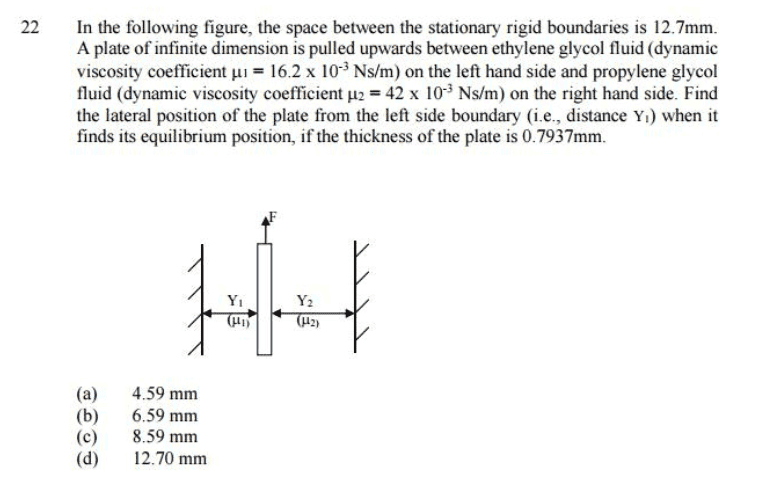# Calculate position of plate between two fluids

• bill222

## Homework Statement

Problem is as shown in the picture.The answer is meant to be a 4.59mm.

Tau=mu(du/dy)

## The Attempt at a Solution

Force (tau) acting on plate from both fluids is equal, as is velocity (du).

mu1 (du/dy1) = mu2 (du/dy2)

mu1/dy1 = mu2/dy2
(mu2/mu1) *y1 = y2

y1 + y2 + plate thickness = width
y1 + y2 + 0.7937 = 12.7 mm

By substituting in mu2/mu1 *y1 = y2 I obtain the answer y1 = 3.31 mm.

Any help is much appreciated

Who says that the force (or shear stress) exerted by each fluid on the plate has to be the same? It seems to me this problem is under-specified.